Waves Exam1 and Problem Solutions

Waves Exam1 and  Problem Solutions

1.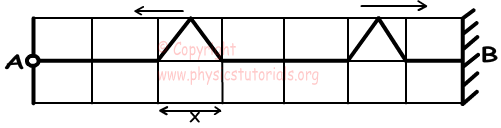Pulses move distance X in one second.Find when they interfere?

Pulse in left hand side of the water tank reflects from open end obstacle and pulse in right hand side of the water tank reflects from fixed end obstacle. After 5,5 seconds later they interfere.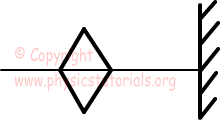2. Two different spring having different densities are added at point B. If the pulse send from one of the spring is reflected and transmitted from point B,  find whether the following statements are true or false. (L1<L2)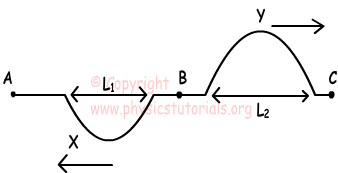I. Y is reflected pulse

II. Density of AB spring is smaller than density of BC spring

III. Incoming wave comes from AB.

Velocity of the pulse in denser spring is smaller than the velocity of pulse in lighter spring. Since, L1<L2, AB is the denser spring so, II is false. Since shapes of pulses  are different we can say that some part of incoming wave is reflected and some part of it is transmitted. I is true and III is false

3. If distance between 5 crests is 60 cm and frequency of the wave source is 3s-1, find velocity of wave.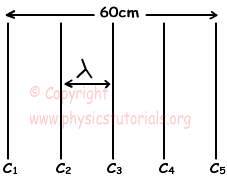4λ=distance between 5crests

4λ=60cm

λ=15cm

λ.f=V

15.3=V

V=45cm/s

4. If the waves A and B coming from point O, to the curved surface, they reflect as shown in the picture given below. Find whether the following statements are true or false.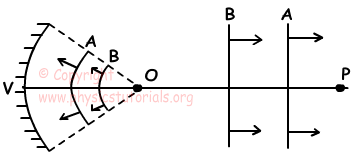I. O is center of the curved surface

II. O is focal point of the curved surface

III. P is the center of the curved surface

Since waves coming from point O, reflects from the curved surface and move parallel, point O becomes focal point of the curved surface. I is false and II is true.

We can not say anything about the center of the curved surface, thus III may be true or false.

5. Which ones of the following statements change wavelength of the wave.

I. Changing frequency of wave source

II. Moving wave source

III. Changing water level of tank

Three of the given statements, given above, change wavelength of wave.

Author: